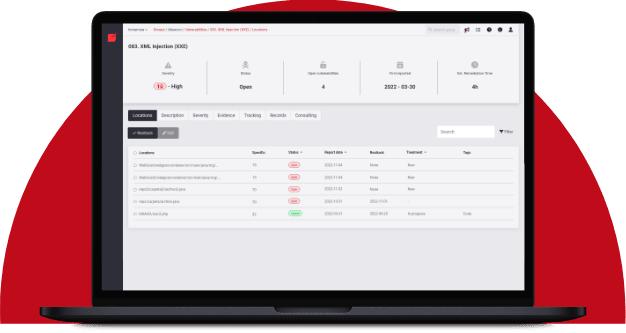## How Bayes Rule affects riskRafael Ballestas

March 5, 2019

Usually, changing our beliefs is seen as a negative thing. But when those beliefs represent our state of uncertainty regarding a particular cybersecurity risk, you’d better use all the tools at hand to reduce that uncertainty, i.e., measuring.

Why do we speak of "belief" and not "probability" here? Intuitively, when we have mentioned probabilities, we’re meaning some belief or measure of uncertainty. For example, when giving a confidence interval, we say we believe the actual value is between the boundaries of the interval, up to a certain degree of confidence. When we simulate multiple scenarios in Monte Carlo simulations and finally aggregate the results, we’re expressing that we believe that the loss will be so many millions or larger.

In science, hypotheses are disproven trough observable, measurable evidence. Similarly, testing in general —and pentesting in particular— can change our beliefs, that is, our initially proposed or prior probabilities, based upon evidence. The mathematical tool for updating these beliefs is a simple one: Bayes Rule. However, it does require us to discuss a few basic probability theory facts. If you’re familiar with it, feel free to skip straight to application to cybersecurity risk.

## Mathematical interlude

Let us consider a simple example for illustrating the basic rules of probability: we have a bag with 2 blue marbles and 3 red ones, and we’re going to draw marbles from the bag (without looking!) and we want to find the probabilities of drawing each kind. Let us call R the event of picking a red marble and B for picking a red one. Their probabilities are P(B) = 2/5, and P(R) = 3/5, in principle.

What if now we draw a second marble? Now the probabilities are subject to the result of the first draw. For example, if we’re given that the first marble picked was blue, then the probability of drawing a red marble is now 3/4 since now there are only 4 balls altogether. This is a conditional probability; it is the chance of event R subject given B happened, denoted P(R|B). This situation can be illustrated with a tree diagram like this:Figure 1. Probability tree diagram. Via MathsIsFun

We can find the probability of a branch, that is, of the succession of two events, by multiplying the probabilities on the arrows, as seen above. And we can add related branches to make up single events: The probability of the third branch from top to bottom is 30%, so if we add that to the previous result, we get that the probability of the second marble being red is 40%. This is an application of the total probability theorem.

We know the conditional probabilities for the second marble given the first, but what if they show us that the second one is blue and we had to guess what the first one was? That’s where Bayes Rule  comes in: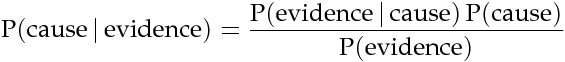Figure 2. Bayes Cause Evidence

If we think of the first event as the cause and the second one as the effect, we have that P(evidence) = 40%. We know that the a priori chance of the first ball being red is 60%, and the probability of observing the evidence given the cause, i.e., P(B|R) = 50%. Hence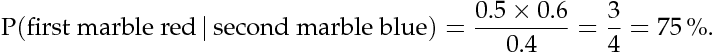Figure 3. First given second

Notice how the extra piece of information, namely that the second marble is blue, narrows down the chance of the first marble being red from the prior probability of 60% to 75%. Hence the probability of the first being blue is the remaining 25%. So I would bet on the first one being red, and I would give you 3 to 1 odds.

This is the power of Bayes Rule: observable evidence, whose likelihood generally depends on the assumed probabilities of the causes, can update or refine our estimates on the likelihoods of the causes.

## So how does this apply to cyber risk?

Since Bayes Rule helps us reduce our uncertainty, it works as a measurement technique. While our initial estimates about an event such as suffering a denial of service or data breach may be way off, we can still get a measurement with those bad estimates, plus evidence, plus their probabilities.

Consider the following random events:

• V: there is a critical vulnerability leading to remote code execution,

• A: suffering a successful denial of service attack (in a reasonable time period v.g. a year)

• T: penetration test results are positive, indicating the possibility of critical vulnerabilities.

Normally, the chain of events here would be that a positive pen test points to the existence of vulnerabilities, and such a vulnerability might lead to the threat (in this case, the denial of service) materializing. Suppose that we know, from the false positive rate, the probability of the existence of vulnerabilities based on a positive and negative pen test, i.e., P(V|T) and P(V|~T). Here the ~ symbol denotes an event not happening.

Now, the existence of a vulnerability does not necessarily imply that the organization will suffer an attack so we might estimate the probabilities of an attack in the case vulnerabilities exist and in the case they don’t. Let P(A|V) = 25% and P(A|~V) = 1%. This, together with P(T) = 1%, the a priori probability that a given penetration test will yield positive results (which we may estimate based on historical data), is all we need to know in order to estimate the posterior probabilities for V, A, and, in fact, anything we might ask about this particular situation.

We might draw a tree diagram like this to describe the situation: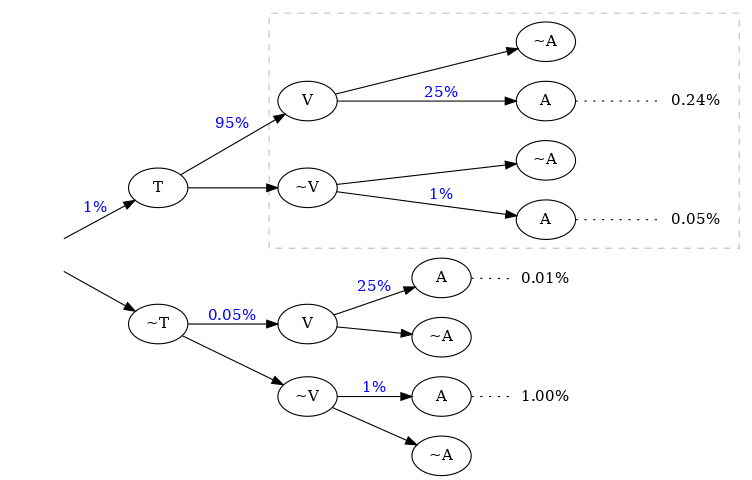Figure 4. Probability tree cyber

Probabilities in blue are the given ones. Since branching in a probability tree implies that the involved probabilities are complementary, i.e., they add up to one, we can compute all others, but we chose not to write them in the above diagram to keep it tidy. Recall that the probability of a single branch is the product of the probabilities that lead to it so we can compute the probabilities of every branch that ends in A, and add them so that P(A) = 1.3%.

If the pen test is positive, what is the probability of being attacked? We could fiddle with formulas, but it’s easier to just look at the subtree after the T, the part of the tree that is framed above. In that case, we have shorter branches ending in A: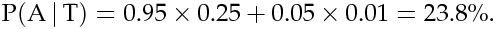Figure 5. Attack Positive Test

What if it is negative?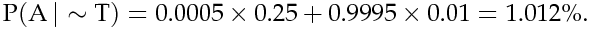Figure 6. Attack Negative Test

Whatever its results, penetration testing gives you more information about the risk your organization is facing. It is especially remarkable that the initial estimate of 1.3% goes up by more than 18 times when the test is positive.

Suppose a year passed, and no denial of service attack happened. Does that mean there are no vulnerabilities? We know the probabilities of attack given the existence of vulnerabilities, but not the other way around. First, we find P(V) by total probability (ignoring all the A nodes in the third column):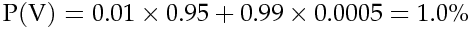Figure 7. Probability of Vulnerabilies

We already know that P(A) = 1.3%, so P(~A) = 98.7%. Finally, by Bayes Rule: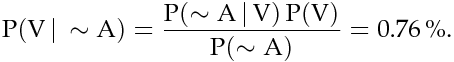Figure 8. Bayes Rule

So even it the threat does not materialize, there is still a latent risk of having vulnerabilities.

This is yet another example of how we can measure risk, even when our initial estimates are bad, using basic probability theory facts and an appropriate decomposition of the problem. We can estimate the probabilities of events given certain assumed conditions, put that together in a probability tree diagram and use the tools learned in this article to generate the rest.

## References

1. D. Hubbard, R. Seiersen (2016). How to measure anything in cibersecurity risk. Wiley.

2. D. Lindley (2006). Understanding Uncertainty. Wiley.

Share

Tags:

## Recommended blog posts

You might be interested in the following related posts.Get an overview of vulnerability assessmentBenefits of continuous over point-in-time pentestingFor which security standards is pentesting a must-have?Pentesting is a system-agnostic approach to securityInjecting JS into one site is harmful, into all, lethalDifferences between these security testing approachesHow BAS solutions work, their importance and benefitsDisclosure rules proposed by SEC may soon take effect

## Start your 21-day free trial

Discover benefits of our Continuous Hacking solution, which hundreds of organizations are already enjoying.